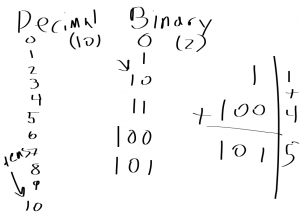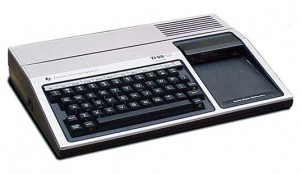# Homeschool Technology Lesson 2: Binary (Continued)

The second homeschool technology lesson was largely a reminder of the first – I reviewed binary. I wrote on the “board” (iPad connected to a TV) decimal numerals…

## Homeschool TechnologyThen for binary, base-2, the first column goes up to… 2 minus 1: 1. Then add a columnI explained again, slowly and repeatedly that decimal, which is base-10, the first column goes up to 10 minus 1: 9. Then you have to add a column. Repeat that over
and over.

Q: For base-5, what does the first column go up to?

A: 4

When you add that second column in base-10, it’s worth ten. So when you add the second column in base-2, it’s worth 2! You got it! So this is lesson 10 (binary)!

Q: For base-8, what is the second column worth?

A: 8

Each column in base-10 from there is ten multiplied by the previous column. So the second column is 10 times the first column number (1): 1×10=10.

The third column is 10 times the value of the second column (10) so 10×10=100.

1×10=10, 10×10=100, 100×10=1000, etc.

10 – 100 – 1000 – 10000, etc.

Same for base-2 – multiply by 2: 2, 4, 8, 16, 32, 64, 128, 256, 512, 1024, 2048, etc.

1 (one), 10 (two), 100 (four), 1000 (eight), 10000 (sixteen), 100000 (thirty-two), etc.

1 (binary, one) plus 100 (binary, four) is 101 (five).

Counting in binary goes:

0 (zero)

1 (one)

10 (add column which is two)

11 (three)

Side note: When I was first learning computer science, I learned from reading manuals thatcame with my TI-99/4A with Extended BASIC. I’d read about a BASIC statement (e.g., if-then) and then write something using it.